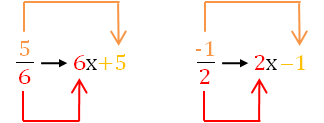## Factoring ax² + bx + c, part 2

In my last post, I showed you a procedure for how to factor a quadratic when the leading coefficient is not equal to 1. Here I refine that method slightly with a short cut that makes your work even quicker. However, this method is a bit more difficult—if you are not really good at mental calculations, you will need to practice this a few times to get it down. To compare methods, I’ll use the same quadratic we factored in the last post.

Problem: Factor$12x^2+4x-5$

1. Our first step is the same as in the previous method—we need to find two numbers that multiply together to give$60$ (that’s$12*-5$) and add together to give$+4$. Of course, those numbers are the same as they were in the last post:$+10$ and$-6$.
……
2. Now here’s the shortcut. Divide both of these numbers by the leading coefficient,$12$. That gives two fractions:
…..
…..$\dfrac{10}{12} \;\; \text{and } \dfrac{-6}{12}$
…..
3. Next, simplify the fractions if possible. In our example, we get
…..
…..$\dfrac{5}{6} \;\; \text{and } \dfrac{-1}{2}$
…..
4. In each fraction, the denominator is the coefficient of the x term in our factor and the numerator is the constant term. So our two factors must be$6x+5$ and$2x-1$. See the diagram below:Blue Taste Theme created by Jabox